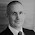## Sunday, August 3

### Pandas 0.14.1

I have just upgraded to Pandas 0.14.1.

It was a pain. At first, none of my graphics programs worked. It looks like a change to the API for parsing Microsoft Excel files was the problem. I am not sure whether my previous approach was wrong (but worked serendipitously), or the API was deliberately changed to break old code (an unusual practice for a point release). If someone knows, I'd appreciate something in the comments below.

What follows are the classes I use to upload Australian Bureau of Statistics (ABS) and Reserve Bank of Australia (RBA) data, with the updates to the parsing stage commented.

And yes, I use Python 2.7, not Python 3 (it's what comes with the Apple Mac).

```### ABSExcelLoader.py
### written in python 2.7 and pandas 0.14.1

import pandas as pd
assert( pd.__version__ >= '0.14.1' )

class ABSExcelLoader:

def load(self, pathName, freq='M', index_name=None, verbose=False):
"""return a dictionary of pandas DataFrame objects for
each Data work sheet in an ABS Excel spreadsheet"""

wb = pd.ExcelFile(pathName)
returnDict = {}

for name in wb.sheet_names:
if not 'Data' in name:
continue

# ExcelFile.parse: API behaviour change with pandas 14.1
#df = wb.parse(sheetname=name, skiprows=8, header=9, index_col=0, na_values=['', '-', ' '])
df = wb.parse(sheetname=name, skiprows=9, header=0, index_col=0, na_values=['', '-', ' '])

periods = pd.PeriodIndex(pd.Series(df.index), freq=freq)
df.set_index(keys=periods, inplace=True)
df.index.name = index_name
returnDict[name] = df

if verbose:
print ("\nFile: '{}', sheet: '{}'".format(pathName, name))
print (df.iloc[:min(5, len(df)), :min(5, len(df.columns))])

return returnDict
```

```### ABSExcelLoader.py
### written in python 2.7 and pandas 0.14.1

import pandas as pd
assert( pd.__version__ >= '0.14.1' )

class RBAExcelLoader:

def load(self, pathName, freq='M', index_name=None, verbose=False):
"""return a pandas DataFrame for an RBA Excel spreadsheet"""
wb = pd.ExcelFile(pathName)
sheetname = 'Data'

# ExcelFile.parse: API behaviour change with pandas 14.1
#df = wb.parse(sheetname, skiprows=9, header=10, index_col=0, na_values=['', '-', ' '])
df = wb.parse(sheetname, skiprows=10, header=0, index_col=0, na_values=['', '-', ' '])

periods = pd.PeriodIndex(pd.Series(df.index), freq=freq)
df.set_index(keys=periods, inplace=True)

if verbose:
print "\nFile: '{}', sheet: '{}'".format(pathName, sheetname)
print 'Columns: {}'.format(df.columns.tolist())
print 'Top left hand corner ...'
print '------------------------'
print df.iloc[:min(5, len(df)), :min(5, len(df.columns))]

return df
```

#### 1 comment:

1.I'd only use skiprows or header - not both as header will skip all rows before that anyway.

Can't say why you had a change from 13 to 14 but your "header=" and 'skiprows=' look to be in conflict to.

From the I/O notes:

"The presence of ignored lines might create ambiguities involving line numbers; the parameter header uses row numbers (ignoring commented lines), while skiprows uses line numbers (including commented lines)"

Maybe you got lucky previously.

cheers